## 11.3.3 The Probabilistic Case: POMDPs

Example 11.14 generalizes nicely to the case ofstates. In operations research and artificial intelligence literature, these are generally referred to as partially observable Markov decision processes or POMDPs (pronounced pom dee peez''). For the case of three states, the probabilistic I-space,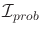, is a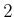-simplex embedded in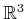. In general, if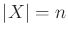, thenis an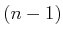-simplex embedded in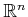. The coordinates of a point are expressed as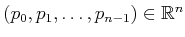. By the axioms of probability,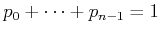, which implies thatis an-dimensional subspace of. The vertices of the simplex correspond to thecases in which the state is known; hence, their coordinates are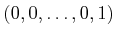,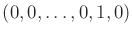,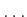,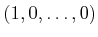. For convenience, the simplex can be projected into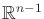by specifying a point infor which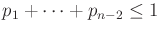and then choosing the final coordinate as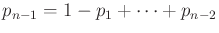. Section 12.1.3 presents algorithms for planning for POMDPs.

Steven M LaValle 2020-08-14